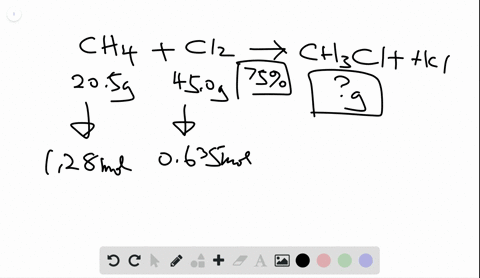🎉 The Study-to-Win Winning Ticket number has been announced! Go to your Tickets dashboard to see if you won! 🎉View Winning Ticket### When 20.5 g of methane and 45.0 g of chlorine gas…

04:18University of Maine
Problem 89

# What is the percent yield of a reaction in which $200 .$ g of phosphorus trichloride reacts with excess water to form 128 $\mathrm{g}$ of $\mathrm{HCl}$ and aqueous phosphorous acid $\left(\mathrm{H}_{3} \mathrm{PO}_{3}\right) ?$

## Discussion

You must be signed in to discuss.

## Video Transcript

25% yield were given information of quantities in reaction as well as amount produced. And we compare how much is produced, which is called the actual yield to how much should be produced. The theoretical yield. And we express this as a percent. And so this is why it's called the percent yield. So, given a reaction with phosphorus, try chloride and water. They react to form hydrochloric acid and h three p 03 We start off with a given quantity of phosphorus, try chloride or 200 grams, and the water is in what's called excess. We produce 128 grams of hydrochloric acid, an additional h threepio for So this is our initial quantity. This is how much we actually produce. So this is the actual yield, and so we need to use our initial quantity of phosphorus. Try chloride to find how much hydrochloric acid should be produced, so we have 200 grams of phosphorus. Try chloride. The question is how many grams of HCL should be produced. It's helpful to create a roadmap to go between these and the very first thing to do even before your roadmap is to write a balanced equation, so phosphorus try chloride. The chemical symbol is P C l three plus water h 20 makes HCL plus H three p 03 So that's art. It's called our skeleton equation. It has all the symbols for each chemical substance, So our next step is to balance the equation. So if I have one phosphorus here and one phosphorus here three Clarins here, one here, two hydrogen, four hydrogen in a total three oxygen's here in one here. I balance it by putting a three here to balance the oxygen's the three here to balance the Koreans. Then everything else is balanced. So my balanced equation is P C L three plus three. It's too Oh, makes three HCL plus H three p 03 Now I can come up with a plan to go from 200 grams of PCL, three two grams of HCL. The first step is to always change your given quantities. Two moles. Once you have moles of the given substance, you can change two moles of the wanted substance and then finally we can change to the quantity we want, which is Ingram's. When we have grams and we want to go to moles, we use the molar mass. So for the first change, I'll use the molar mass of PCL three go from moles of one substance, two moles of the other substance. I need to use the ratio from the balanced equation. And finally, once I have moles to go two grams, I use the molar mass again And this time we for HCL So we have all the information to find out for theoretical yield 200 grams PCL three change two moles I divide by the molar mass of PCL three, which is 137 0.33 grams. I can then go two moles of hydrochloric acid using the coefficients from the balanced equation For every one mole of PCL three, there are three moles of HCL. Finally, I confined the mass of HCL that should be produced using the molar mass One more is equal to 36.46 grams and the molar mass remember comes from the periodic table by adding up the atomic mass of hydrogen plus the atomic mass of chlorine. And so when I multiplied 200 times three times 36.46 and divide by 137.33 I should form 159.30 grams of age. CEO. This is the theoretical yield. How much I should form. So to find my percent you it's the actual quantity that I produced. 128 divided by how much I should have gotten times 100 were 80.4%.